# Stokes formula

(diff) ← Older revision | Latest revision (diff) | Newer revision → (diff)

A formula that expresses the connection between the flow of a vector field through a two-dimensional oriented manifold and the circulation of this field along the correspondingly oriented boundary of this manifold. Letbe an oriented piecewise-smooth surface, let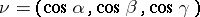be the unit normal to(at those points, of course, where it exists), which defines the orientation of, and let the boundary ofconsist of a finite number of piecewise-smooth contours. The boundary ofis denoted by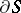, and is oriented by means of the unit tangent vector, such that the orientation ofobtained is compatible with the orientationof.

Ifis a continuously-differentiable vector field in a neighbourhood of, then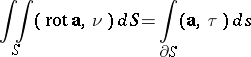(*)

(is the area element of,is the differential of the arc length of the boundaryof) or, in coordinate form,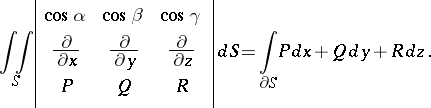Stated by G. Stokes (1854).

Stokes' formula is also the name given to a generalization of formula , which represents the equality between the integral of the exterior differential of a differential formover an oriented compact manifoldand the integral of the formitself along the boundary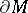of(the orientation ofis taken to be compatible with that of):Other particular cases of this formula are the Newton–Leibniz formula, the Green formulas and the Ostrogradski formula.